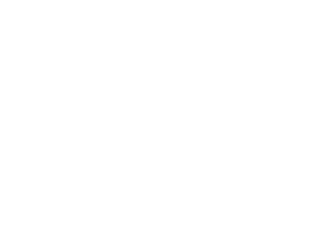DownloadDownload PresentationThe New

# The New

Télécharger la présentation## The New

- - - - - - - - - - - - - - - - - - - - - - - - - - - E N D - - - - - - - - - - - - - - - - - - - - - - - - - - -
##### Presentation Transcript

1. The New Math Family Feud

2. Game 1

3. A triangle has a base of length 13 and the other two sides are equal in length. If the lengths of the sides of the triangle are integers, what is the shortest possible length of a side?

4. P is the set of positive factors of 20 and Q is the set of positive factors of 12. If x is a member of set P and y is a member of set Q, what is the greatest possible value of x - y?

5. In the right triangle ABC below, . What is the value of y?

6. If n is divided by 9, the remainder is 5. What is the remainder if 3n is divided by 9?

7. Let k # j be defined as the sum of all integers between k and j. For example, 5 # 9 = 6 + 7 + 8 = 21. What is the value of (80 # 110) - (81 # 109)?

8. The New Math Family Feud

9. Game 2

10. A rectangular solid has two faces the same size and shape as figure I below and four faces the same size and shape as figure II below. What is the volume of the solid?

11. What is the least number of 2's that can be multiplied together to yield a number greater than 50?

12. In square PQRS below, QR = 1, RU = US, and PT = TS. What is the area of the shaded region?

13. If x2 + y2 = 2xy, then x must equal: